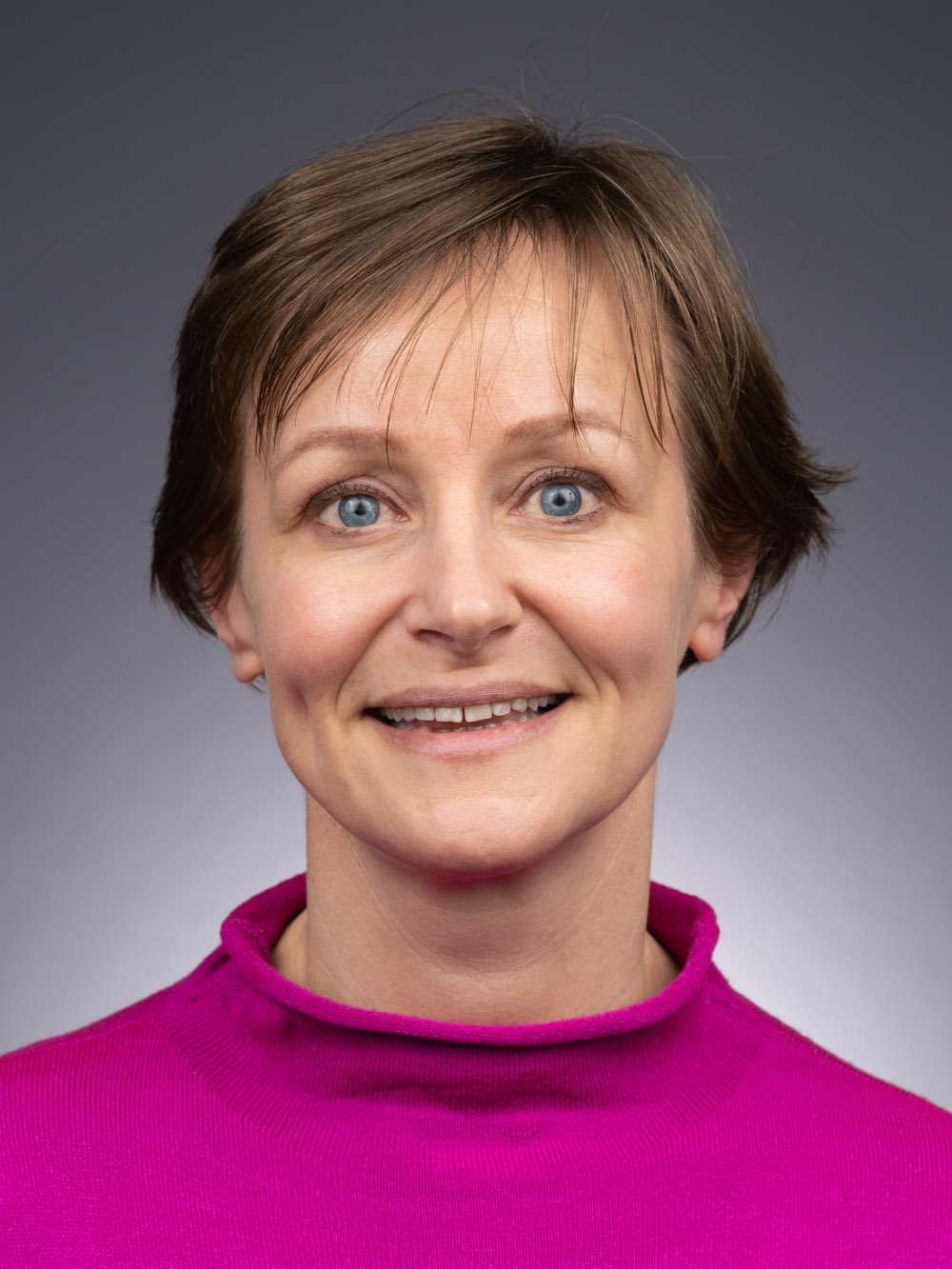Research

### Closing the Gap: Finding Better Algorithms and Better Bounds

My research interests are in combinatorial optimization. I design and analyze algorithms for optimization problems arising in areas such as:

• information science, in particular, aggregating inconsistent structured information;
• network design, scheduling and logistics;
• computational biology.
For many problems of interest, finding optimal solutions is computationally intractable. My work specifically focuses on practical algorithms for approximately solving these problems, by leveraging techniques from:
• discrete mathematics, such as graph theory, combinatorics and matroid theory;
• computer science, such as complexity analysis, datastructures, and approximation algorithms;
• operations research, such as linear programming and network flows.
My work has been supported by a Simons Collaboration Grant for Mathematicians. In addition to theoretical research, I work with undergraduate and graduate students on practical applications of Operations Research. In recent project, we used network optimization to optimize the matching of students and pre-major advisors at William & Mary.

### Publications

#### Permutations: Sorting and Card Shuffling